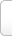﻿ 将组词_将字可以怎么组词_能组什么词_有哪些

# 将 字可以怎么组词

• 将功赎罪
• 将信将疑
• 将迎
• 将阳
• 将傍
• 将于
• 将匠
• 将抚
• 将器
• 将谓
• 将竽
• 将军府
• 将作监
• 将欲取之必先与之
• 将慎
• 将功补过
• 将无做有
• 将星
• 将久
• 将来
• 将官
• 将晚
• 将息
• 将毋
• 将种
• 将然
• 将明
• 将兵长史
• 将夜
• 将错就错
• 将功折过
• 将顺
• 将相本无种
• 将尉
• 将功折罪
• 将护
• 将事
• 将用
• 将礼
• 将将
• 将养
• 将取固予
• 将就
• 将门
• 将在外，主令有所不受
• 将纔
• 将治
• 将送
• 将助
• 将佐
• 将伯之助
• 将次
• 将扶
• 将近
• 将帅
• 将毋同
• 将指
• 将心比心
• 将台
• 将相器
• 将计就计
• 将陋就简
• 将惜
• 将要
• 将摄
• 将牢
• 将欲
• 将略
• 将台堡
• 将伯之呼
• 将闾
• 将美
• 将离
• 将本求财
• 将仕郎
• 将本求利
• 将无同
• 将承
• 将倾
• 将材
• 将门有将
• 将弁
• 将游
• 将作长史
• 将夺固与
• 将在外，君命有所不受
• 将车
• 将丧
• 将军炭
• 将遇良才
• 将身
• 将军
• 将命
• 将吏
• 将机就机
• 将至
• 将妄
• 将类
• 将爷
• 将家
• 将为

## “将”字在中间的能组哪些词

• 行将就木
• 内将军
• 云麾将军
• 棕将军
• 皮之不存﹐毛将安傅
• 尽盘将军
• 国将不国
• 皮之不存﹐毛将焉附
• 潘将军
• 反将计
• 蒲将军
• 上将军
• 人之将死其言也善
• 强将手下无弱兵
• 矬子里头选将军
• 麻将牌
• 人之将死，其言也善
• 日中将昃
• 侯王将相宁有种乎
• 兵来将迎，水来土堰
• 太平本是将军定，不许将军见太平
• 李将军列传
• 兵来将挡，水来土掩
• 鸟之将死，其鸣也哀
• 常将有日思无日，莫待无时思有时
• 兵来将挡水来土掩
• 王侯将相
• 计将安出
• 斑寅将军
• 兵微将寡
• 一将功成万骨枯
• 马将牌
• 铁将军把门
• 蜀中无大将，廖化作先锋
• 铁将军
• 水来土掩，兵到将迎
• 百虫将军
• 斩将刈旗
• 大将军
• 大将风度
• 偏将军
• 洞天圣酒将军
• 仪同大将军
• 丁将军
• 老将行
• 六将军
• 兵败将亡
• 无将大车
• 蟹将虾兵
• 客将军
• 水来土压，兵至将迎
• 器满将覆
• 黑矟将军
• 五威将军
• 霍将军
• 拜将封侯
• 石将军
• 兵强将勇
• 布衣将相
• 物极将返
• 平东将军
• 车骑将军
• 骠骑将军
• 出将入相
• 伊犁将军
• 棋逢对手，将遇良才
• 逝将去汝
• 水来土掩，兵至将迎
• 白将军
• 大厦将颠
• 挈妇将雏
• 五道将军
• 军多将广
• 败军之将，不可言勇
• 金吾将军
• 瘟将军
• 骄将悍卒
• 干将莫邪
• 红将军
• 金华将军
• 大厦将倾
• 捋臂将拳
• 抚军将军
• 鸷鸟将击，卑飞敛翼
• 刘猛将军
• 兵来将敌，水来土堰
• 韩信将兵
• 庾将军
• 兵少将微
• 镇殿将军
• 皮之不存，毛将安傅
• 千军易得，一将难求
• 出入将相
• 皮之不存，毛将焉附
• 草率将事
• 大汉将军
• 军来将敌，水来土堰
• 龙骧将军
• 骠骑大将军
• 独用将军
• 贰师将军

## “将”字在结尾组词有哪些

• 诸将
• 熊虎将
• 败兵折将
• 杨家将
• 牙将
• 诞将
• 登坛拜将
• 横行将
• 五威将
• 寡将
• 谋臣猛将
• 运将
• 举将
• 残军败将
• 健将
• 门将
• 几将
• 破军杀将
• 逸将
• 拘神遣将
• 反将
• 爪牙之将
• 无将
• 出将
• 龙城飞将
• 谢将
• 天将
• 云将
• 虓将
• 我将
• 打麻将
• 参将
• 闯将
• 鸣将
• 分身将
• 肃将
• 五官将
• 列将
• 天兵天将
• 虎将
• 山东山相，山西出将
• 边将
• 猛将
• 将将
• 五星上将
• 故将
• 兵对兵，将对将
• 损军折将
• 干将
• 偏将
• 射鸱都将
• 蹚将
• 贪将
• 嚄唶宿将
• 子将
• 代将
• 区区将将
• 若将
• 冗将
• 耆将
• 戎将
• 阴阳不将
• 以二卵弃干城之将
• 谋臣武将
• 驼鼍将
• 上将
• 走马换将
• 记将
• 败军之将
• 户将
• 佐将
• 哼哈二将
• 神兵天将
• 就将
• 莘莘将将
• 慎将
• 骁将
• 鋭将
• 照将
• 护将
• 天策上将
• 仙将
• 过关斩将
• 少将
• 虾兵蟹将
• 别将
• 输将
• 赠边将
• 马将
• 将门有将
• 次将
• 都将
• 命将
• 手将
• 久将
• 五将
• 突将
• 亚将
• 雄将
• 自将
• 节将

### 将 的拼音及解释

jiāngTip:SCCG